# Introduction

This vignette describes the extenstions to the rtrim package as per version 2.0:

• Monthly data
• Extended plotting of time-totals
• Extended plotting of indices
• Running TRIM in a stratified manner
• Heatmap visualisations of both observed and imputed data

See also the vignettes rtrim confidence intervals and taming overdispersion for additional new features.

# Monthly data

One of the major improvements in rtrim 2.0 with respect to 1.0 is the handling of monthly, or any other intra-annual, data. For example, where a classic TRIM model 3 looks like $\ln \mu_{ij} = \alpha_i + \beta_j$ where $$\mu_{ij}$$ is an expected count, $$\alpha_i$$ is a site parameter for site $$i$$ and $$\beta_j$$ is a time point parameter for year $$j$$. the extension towards months looks like $\ln \mu_{ijm} = \alpha_i + \beta_j + \delta_m$ where $$\delta_m$$ are month parameters for month $$m$$.

Please take a look in Models and statistical methods in rtrim for a full explanation, and application to other model formulations.

The general syntax to specify R-Trim models that use monthly data is as follows:

  z <- trim(count ~ site + year, data=...)                   # simple annual data
z <- trim(count ~ site + year + habitat, data=...)         # using covariates
z <- trim(count ~ site + (year+month), data=...)           # using monthy data
z <- trim(count ~ site + (year+month) + habitat, data=...) # using both

Note the use of brackets to distinguish months from covariates.

Here is a full example for Oystercatcher data, which now comes with rtrim 2.0

rm(list=ls()) # always start with a clean slate
library(rtrim)
data(oystercatcher)
oc <- trim(count ~ site + (year+month), data=oystercatcher, model=3, overdisp=TRUE,
constrain_overdisp=0.999)
#> Warning in trim_estimate(count = count, site = site, year = year, month =
#> month, : Removed 15 sites without positive observations: (1, 30, 41, 48, 66, 69,
#> 78, 82, 83, 85, 86, 87, 88, 92, 104)
plot(index(oc))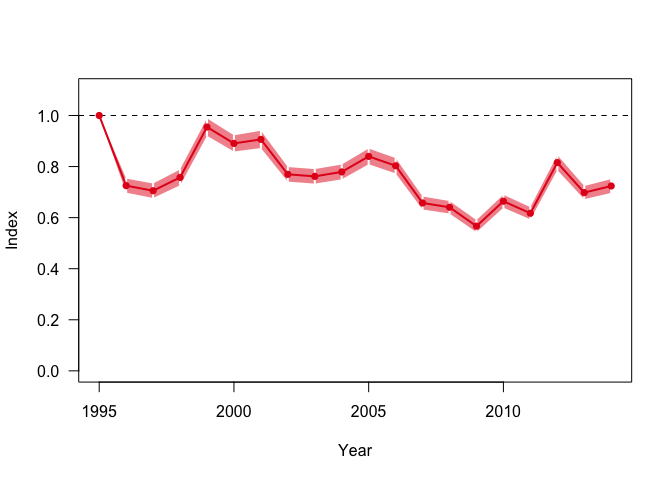## Comparison with UIndex

While in the past TRIM was used to analyse count data with an annual resolution (i.e. one observation per site per year), the software package UIndex [Underhill, 1989; Underhill and Prŷs-Jones, 1995] was and is used to analyse count data with higher (e.g., monthly) resolution. As demonstrated above, rtrim is extended to accept and analyse monthly data as well. This section demonstrates the application of rtrim to monthly data, and compares the output with that of UIndex.

### UIndex

UIndex was used to analyse the monthly Oystercatcher counts, collected by SOVON Netherlands. Here we show the pre-saved output of UIndex, as the main trend, and the 90% consistency intervals’. Note also the use of 2004 as base year.

load("UIndex_Oystercatcher_output.RData")
yrange <- range(uidx$index, uidx$lower, uidx$upper) plot(uidx$year, uidx$index, type='l', xlab="Year", ylab="Index", ylim=yrange) segments(uidx$year, uidx$lower, uidx$year,uidx$upper) legend("topright", "UIndex", col="black", lty="solid") # Add index=1 line for reference abline(h=1.0, lty="dashed", col=gray(0.5)) # Mark the base year ibase <- match(2004, uidx$year)
points(uidx$year[ibase], uidx$index[ibase], pch=16)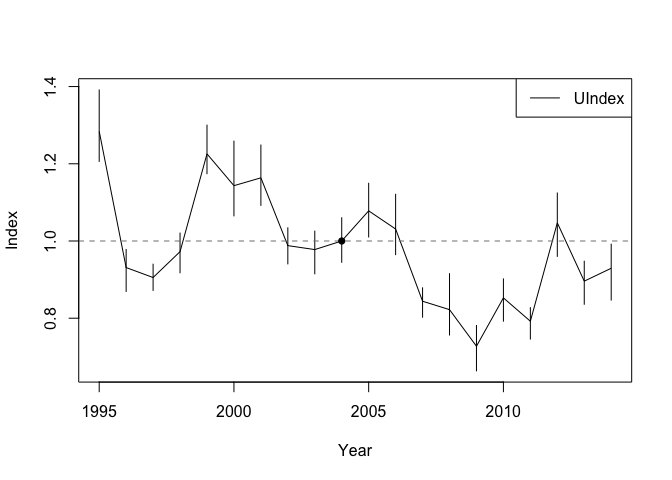### rtrim

Here we show the comparision with rtrim, using the results computed above.

# Compute and plot an index for Oystercatcher counts, using 2004 as base year and
# adding 90% confidence intervals as well.
idx <- index(oc, level=0.9, base=2004)
plot(idx, band="ci")

# Plot UIndex on top
lines(uidx$year, uidx$index)
segments(uidx$year, uidx$lower, uidx$year,uidx$upper, lwd=2)

legend("bottom", c("UIndex","TRIM"), col=c("black","red"), lty="solid")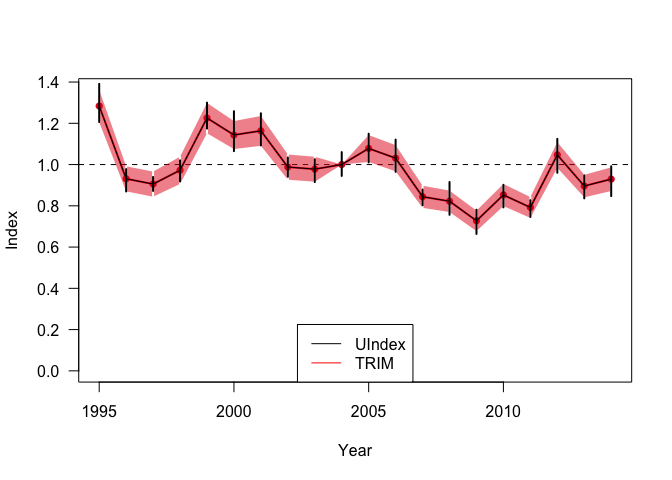Note the computation and display of confidence intervals, which is new for rtrim 2.0, along with the standard errors of both classic TRIM and rtrim 1.0.

This plot demonstrates that the indices as computed by UIndex and rtrim are virtually identical, and that the 90% confidence intervals of TRIM are well comparable to the 90% consistency intervals of TRIM, although both are estimated using completely different approaches. In the case of TRIM, confidence intervals are based on standard errors which are derived analytically as part of the GEE estimation process and ultimately are based on the variance within the orginal data. See the vignettes Models and statistical methods in rtrim and rtrim confidence intervals for more information. Consistency intervals in UIndex are estimated by means of a bootstrap method. See Underhill  and Underhill and Prŷs-Jones  for more information.

# Stratified rtrim

Sometimes it can be usefull to combine rtrim results for different regions (‘strata’) into a single, larger scale (‘superstratum’) rtrim analysis. One particular example is the case where individual EU countries use TRIM or rtrim to compute indices for their own countries, and submit the results to the European Bird Census Counsil EBCC for aggregatation on the EU scale, see van Strien et al.  for an example using the previous stand-alone version of TRIM. In this case, the output of the lower scale rtrim runs, i.e., the time totals, are used as ‘observations’ in the higher scale run. Although this procedure works out well for the estimates and indices, it doesn’t work for the associated standard errors, because the time totals are not Poisson distributed, where the original counts are. To circumvent this problem, rtrim has options to export the variances of the lower scale runs and to import these into the higher scale runs, to use instead.

The following example shows the associated workflow. Strictly for demonstration purposes, we split the Skylark dataset into two ‘regions’ associated with the habitats (heath and dunes).

# split data
data(skylark2)
heath <- subset(skylark2, habitat=="heath") # 208 records
dunes <- subset(skylark2, habitat=="dunes") # 232 records

heath$site <- factor(heath$site) # get rid of empty levels
dunes$site <- factor(dunes$site)

# run models
m1 <- trim(count ~ site + year, data=heath, model=3)
m2 <- trim(count ~ site + year, data=dunes, model=3)

# collect imputed time-totals (which is the default)
t1 <- totals(m1)
t2 <- totals(m2)

plot(t1,t2, names=c("heath", "dunes"))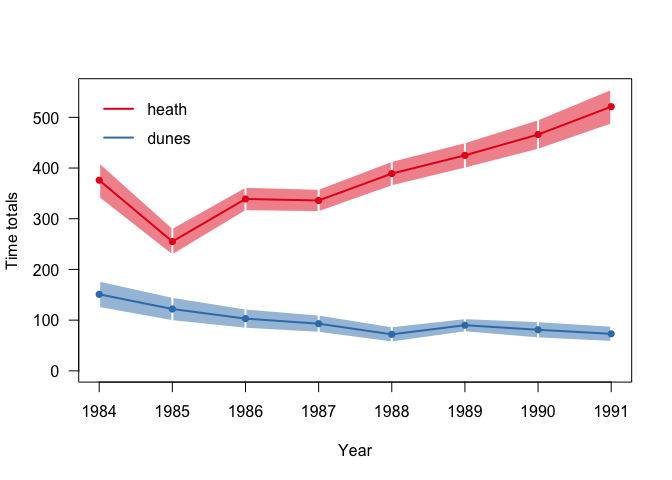Note the use of multiple time-totals in a single plot (new for rtrim 2.0)

The next step is to use the time totals for the differente habitats (strata') as input data for an upscaled (superstratum’) run. The habitat types now serve as site names, and imputed counts will be the input counts.

t1$region <- "heath" t2$region <- "dunes"
t12 <- rbind(t1, t2)
#>   time imputed se_imp region
#> 1 1984     376     33  heath
#> 2 1985     255     25  heath
#> 3 1986     339     22  heath
#> 4 1987     336     21  heath
#> 5 1988     389     23  heath
#> 6 1989     425     24  heath

The final preparation step is to extract the variance-covariance information for the different habitats, and combine them into a single list, using habitat/region names as identifier, enabling the correct match between the site identifiers in the data, and the variance-covariance matrices.

# Also collect the variance-covariance matrices for both runs
vcv1 <- vcov(m1)
vcv2 <- vcov(m2)
vcv3 <- list(heath=vcv1, dunes=vcv2)

and off we go with the superstratum run. Note the new argument covin to use the variance-covariance data.

m3 <- trim(imputed ~ region + time, data=t12, model=3, covin=vcv3)
plot(totals(m3))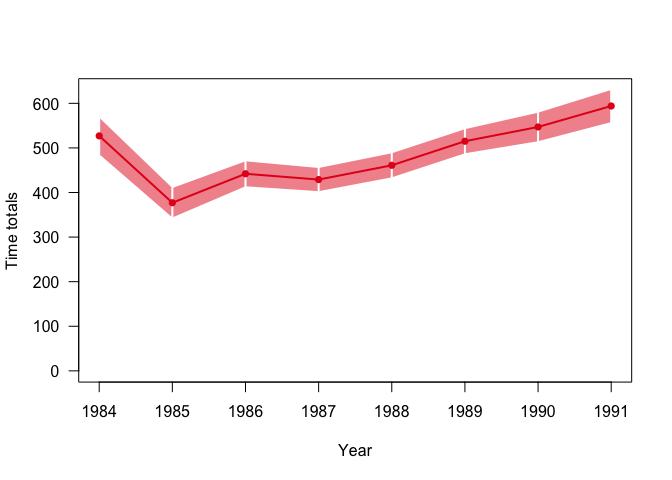Now, just for comparison, we compare index plots for both the baseline run (where dunes and heath are taken together, but do act as covariates) and the upscaled superstratum’ variant.

m0 <- trim(count ~ site + year + habitat, data=skylark2, model=3) # baseline
t0 <- totals(m0)
t3 <- totals(m3)
plot(t0,t3, names=c("baseline","superstrata"))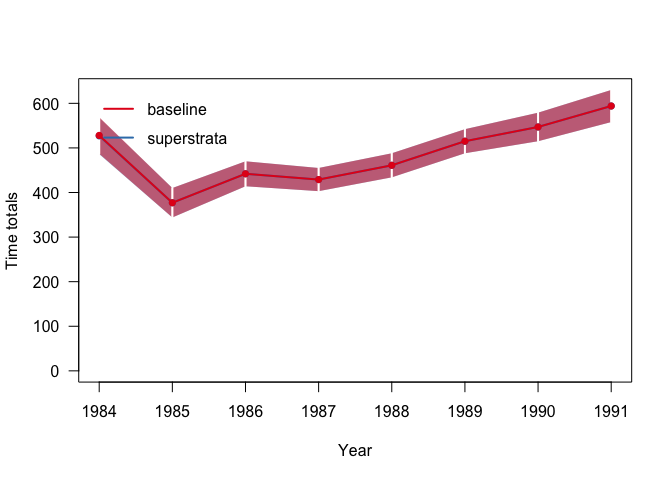Which suggests that for this example the differences are small, if any.

# Taming overdispersion

In some cases, especially with clustering bird species, overdispersion can be huge, reaching unrealistic values of more than 500. rtrim now contains an option to constrain the computed value of overdispersion by detecting outliers, and removing them from the computation of overdispersion (but retaining them for all other calculations). The full rationale and methdology is described in Taming overdispersion, but the actual use is rather simple.

Take for example the Oystercatcher data, which results in a huge overdispersion of about 850

data(oystercatcher)
m1 <- trim(count ~ site + (year + month), data=oystercatcher, model=3, overdisp=TRUE)
#> Warning in trim_estimate(count = count, site = site, year = year, month =
#> month, : Removed 15 sites without positive observations: (1, 30, 41, 48, 66, 69,
#> 78, 82, 83, 85, 86, 87, 88, 92, 104)
overdispersion(m1)
#>  850.7956

The inclusion of the option constrain_overdisp=0.999 triggers the detection of outliers that have a probability of 0.1%.

m2 <- trim(count ~ site + (year + month), data=oystercatcher, model=3, overdisp=TRUE,
constrain_overdisp=0.99)
#> Warning in trim_estimate(count = count, site = site, year = year, month =
#> month, : Removed 15 sites without positive observations: (1, 30, 41, 48, 66, 69,
#> 78, 82, 83, 85, 86, 87, 88, 92, 104)
overdispersion(m2)
#>  102.6988

And so we get a much more reasonable result, with smaller standard errors.

t1 <- totals(m1)
t2 <- totals(m2)
plot(t1, t2, names=c("unconstrained","constrained"), leg.pos="bottom")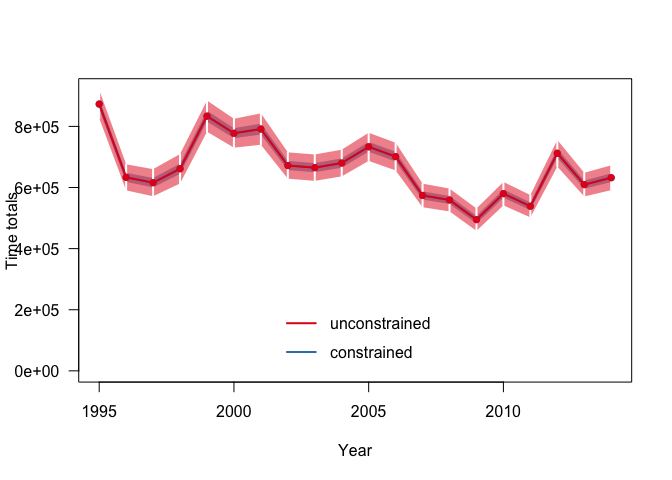# Output visualization

## Plotting time-totals

Once an rtrim model has been estimated, one of the first steps of analysis schould be the plotting of time-totals. This is done by first calling the totals() function, and then a custom plot() function:

rm(list=ls())                          # New section; time for a new blank slate
data(skylark2)                                             # reload Skylark data
m1 <- trim(count ~ site + year, data=skylark2, model=3)
t1 <- totals(m1)          # By default, the time-totals for the imputed data set
plot(t1)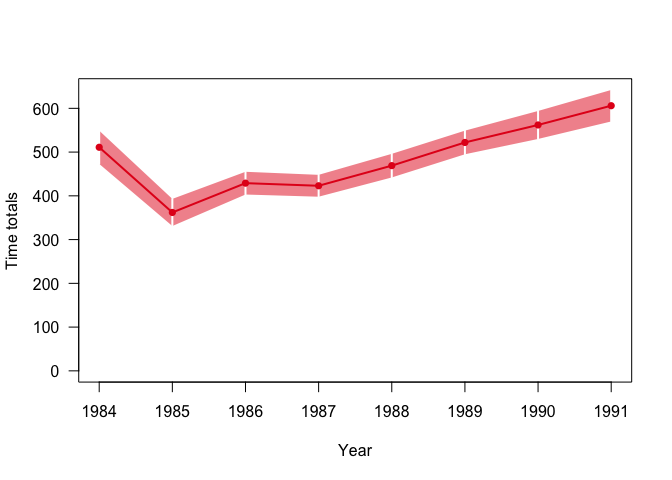Alternatively, one may compute the fitted time-totals. the next example shows the plotting of both the imputed and fitted time-totals, and also demonstrates how series can be named, and the plot can be decorated with a main title.

m2 <- trim(count ~ site + year, data=skylark2, model=2, changepoints=c(1,2))
ti <- totals(m2, "imputed")
tf <- totals(m2, "fitted")
plot(ti, tf, names=c("imputed","fitted"), main="Skylark", leg.pos="bottomright")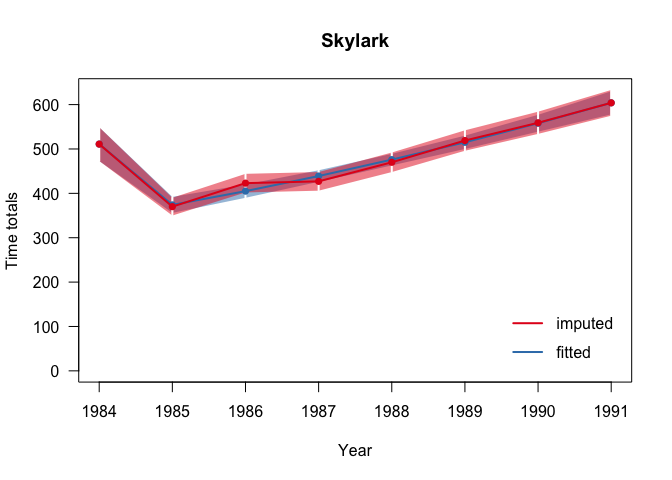Since imputed totals are composed of both observed and estimated counts, it might be insightful to plot the observed counts as well.

m3 <- trim(count ~ site + year, data=skylark2, model=3)
t3 <- totals(m3, obs=TRUE)          # Extract observations in addition to totals
plot(t3)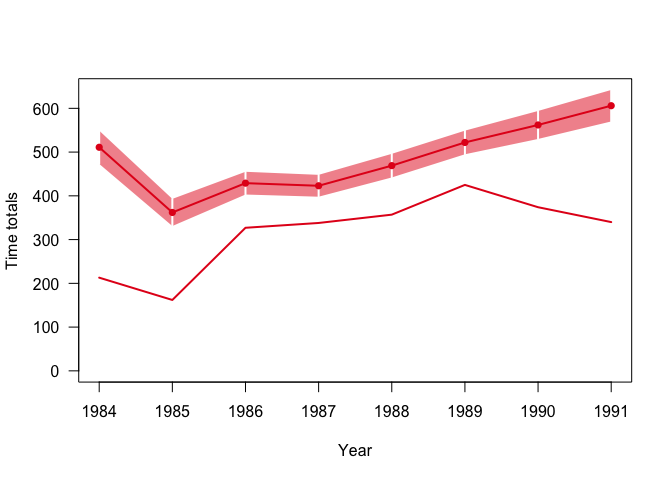As can be seen, the amount of observed Skylarks is considerable smaller than the time totals suggest. Futhermore, it can be seen that while the observed counts decrease from 1989, the imputed counts continue to increase. It is thus suggested to look into more detail what is going on in different sites.

## Plotting indices

Once a TRIM model has been estimated, and indices are computed, these latter can be plotted using the generic plot command plot() (which, behind the screens, calls plot.trim.index()).

m <- trim(count ~ site + year, data=skylark2, model=3) # Run a fairly basic TRIM model
idx <- index(m) # By default, the indices for the imputed data set
plot(idx)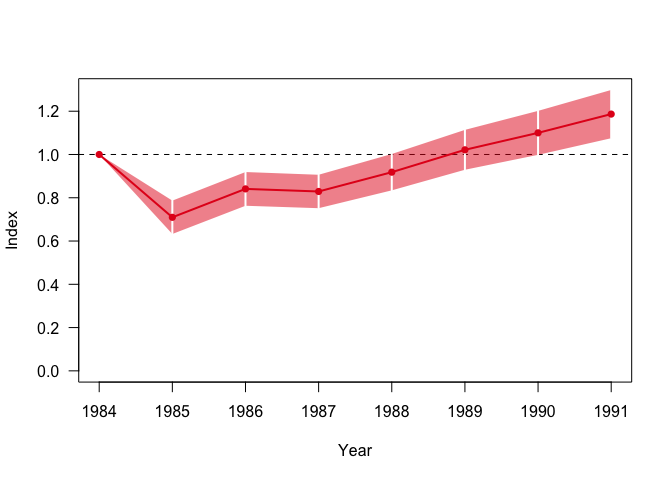If required, the x-axis and y-axis labels as well as the tile can be defined, and the index can be expressed as a percentage, instead as a fraction. This example shows all these options:

plot(idx, xlab="Year AD", ylab="Index (%)", main="Skylark index", pct=TRUE)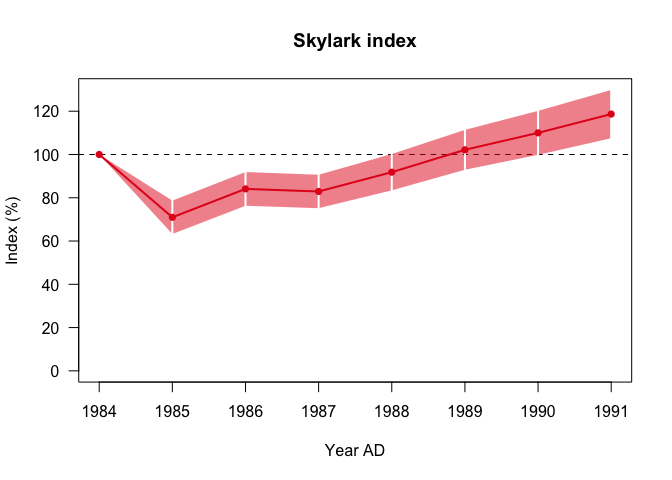### Indices and covariates

When covariates are involved, it can be helpful to compute and plot indices for the various covariate categories as well. The next example demonstrates this.

m <- trim(count ~ site + year + habitat, data=skylark2, model=3) # Run a fairly basic TRIM model
idx <- index(m, covars=TRUE)
plot(idx)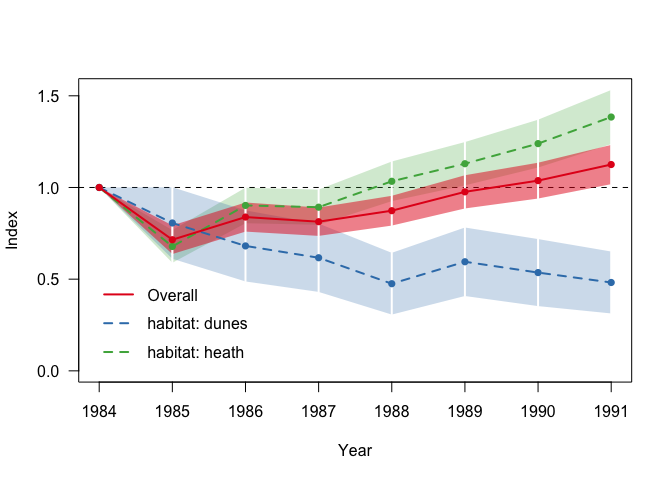As can be seen, indices for the various covariate categories are automatically plotted as well. This behaviour can be supressed by setting covar="none" in the call to plot() (note the use of plural covars' in the call toindex()--- because indices for multiple covariates can be computed, and the singularcovarin the call toplot() — because only one of them can be used for a single figure)

### Combining multiple indices

Indices for multiple TRIM runs can be combined in a single plot.

data(skylark2)
m0 = trim(count ~ site + year          , data=skylark2, model=3)
m1 = trim(count ~ site + year + habitat, data=skylark2, model=3)

idx0 <- index(m0)
idx1 <- index(m1)

plot(idx0, idx1)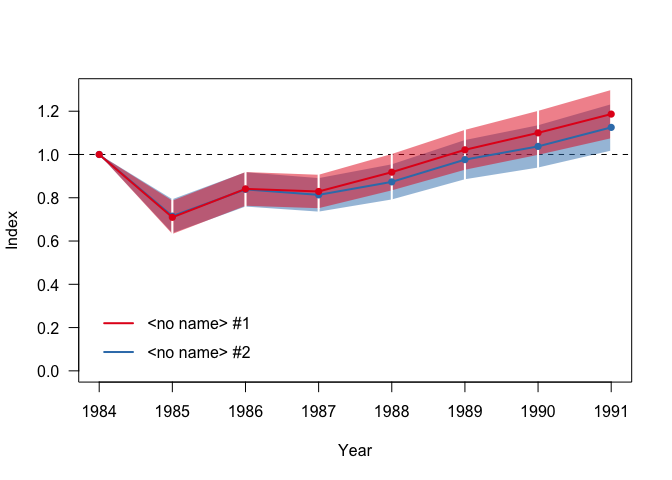As you see, a legend is inserted automatically. You can change the names of the series by using the names argument:

plot(idx0, idx1, names=c("Without covariates", "Using 'Habitat' as covariate"))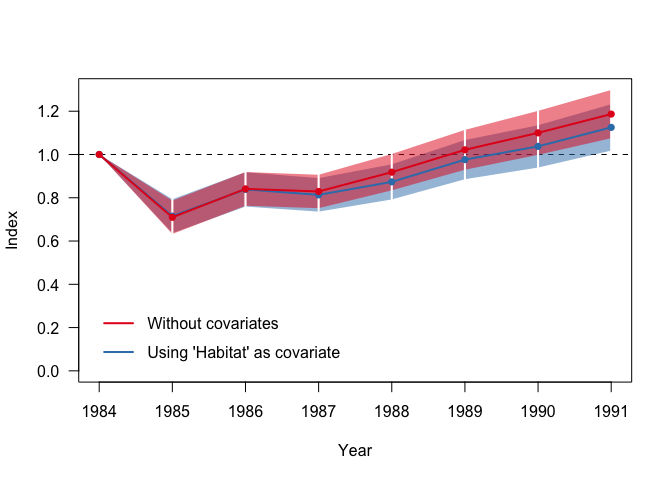## Adding confidence intervals.

New in rtrim 2.0 is the possibility to express uncertainty as a confidence interval, in addition to the standard errors. Both the functions totals() and index() now accept the option level that specifies the confidence level and triggers the computation.

m <- trim(count ~ site + year, data=skylark2, model=3)
tt <- totals(m, level=0.95)                   # Compute 95% confidence intervals
#>   time imputed se_imp       lo       hi
#> 1 1984     511     38 434.9422 583.8739
#> 2 1985     362     31 299.7129 421.2013
#> 3 1986     429     26 376.8626 478.7599
#> 4 1987     423     25 372.8600 470.8378
#> 5 1988     469     27 414.9102 520.7285
#> 6 1989     522     27 467.9707 573.7910

So, the lower and upper bounds of the confidence interval is stored in columns lo and hi. These are automatically picked up by the plot() function.

plot(tt)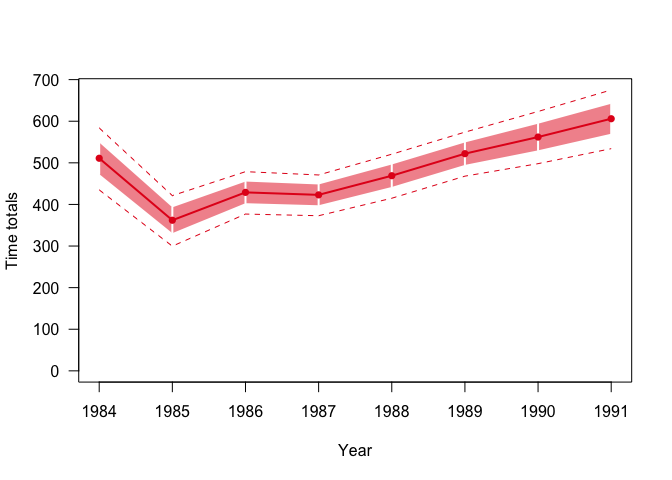If required, the uncertainty band, which is by default plotted using standard errors, can be plotted using the confidence intervals when the option band="ci" is used.

plot(tt, band="ci")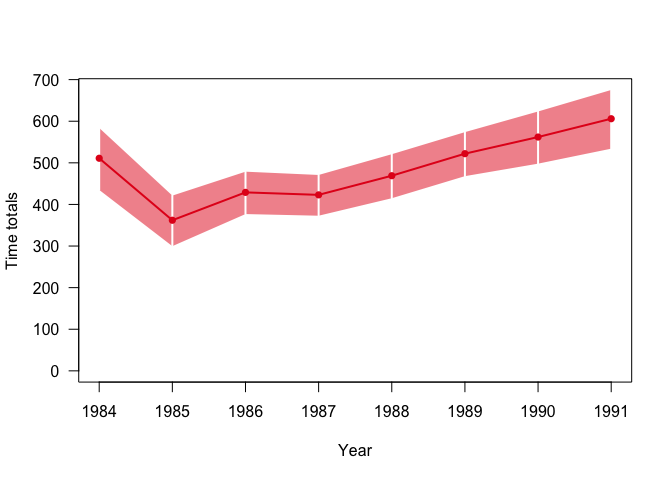See vignette rtrim confidence intervals for more information on the underlying methodology.

## Plotting heatmaps.

The detailed spatiotemporal structure of both the observed and the imputed data can be inspected by means of the function heatmap() that operates on the output of trim(). The default behaviour of this function is to display a heat map of the observed counts only:

m <- trim(count ~ site + year, data=skylark2, model=3)
heatmap(m, main="Skylark, observations")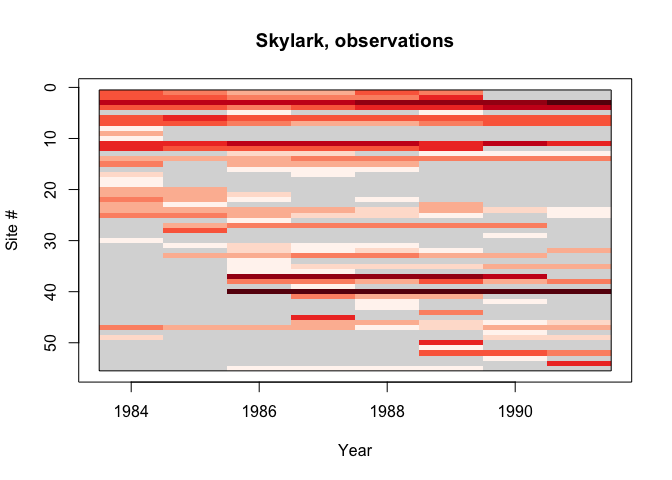In this heatmap, site/time combinations are colored by (log) counts: lower counts are colored a pale red, and high counts a dark red. Consistent with the nature of count data, this color scale is proportional to the log counts. Observed counts of 0 cannot be represented this way and are colored white. Missing site/time combinations are marked as gray.

It can be seen that the observational coverage is not constant: most sites have incomplete records, especially in the earlier years. This is a typical patern for an expanding observation program, but may have consequences for the statistical analysis, because the imputation for these years will in fact be an extrapolation back in time.

The next example shows the TRIM estimated counts (using shades of blue, rather than red:

heatmap(m, "fitted", main="Skylark, TRIM estimates")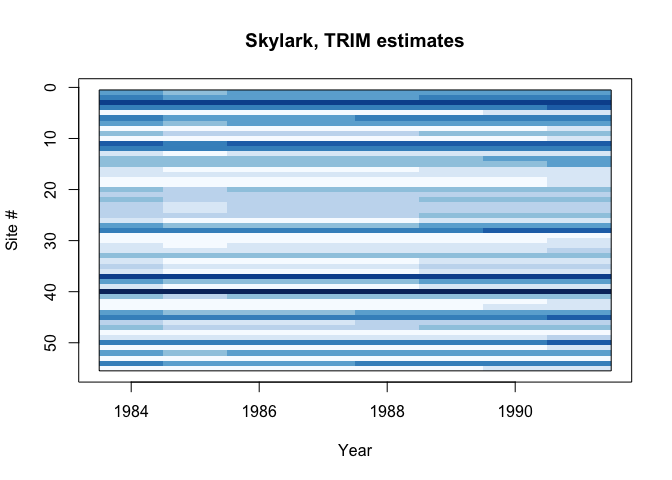From this plot, it is clear that the variance between sites is much higher than the variance between years. In fact, the trend in time can hardly be seen.

The last example sows the heatmap for the imputed data, where estimates are used to fill up the missing observations. Again, red is for obervations, blue for estimates.

heatmap(m, "imputed", main="Skylark, imputed data")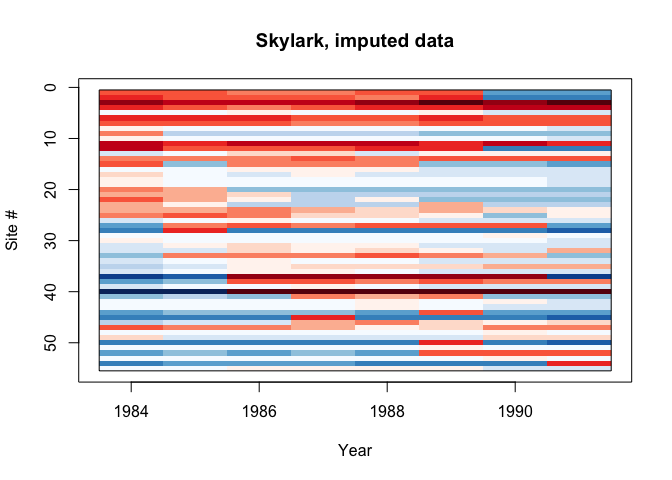### Heatmaps for monthly datasets

For monthly data, heatmaps work slightly different, but in the same spirit:

data(oystercatcher)
m <- trim(count ~ site + (year + month), data=oystercatcher, model=3, overdisp=TRUE)
#> Warning in trim_estimate(count = count, site = site, year = year, month =
#> month, : Removed 15 sites without positive observations: (1, 30, 41, 48, 66, 69,
#> 78, 82, 83, 85, 86, 87, 88, 92, 104)
heatmap(m, "imputed", main="Oystercatcher (imputed)")`